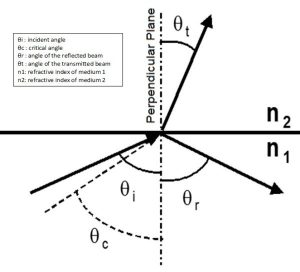# The role of evanescent field in optical fiber sensing

In this post we will briefly go through a theoretical introduction of evanescent field associated to optical fiber sensors and its applications to perform absorption measurements.

First, it is important to introduce the term of total internal reflection (TIR). TIR, consists of an optical phenomenon that occurs when a light beam hits the interface between two media with an angle greater than the critical angle (θc) with respect to the plane perpendicular to the surface as it is shown in the figure below.Figure 1. Reflection and refraction phenomena at the interface between two media with different refractive index.

This critical angle (θc) that marks the limit in which the transmission through TIR will occur or not, is given by eq. (1), where n2/n1 is given in radians.

(1)

A special design case for light guidance consists of optical fibers. Here, the refractive index of the optical fiber core is greater than that of the optical fiber cladding (n1> n2), which facilitates the guidance of the light that couples into the fiber by means of TIR. Despite the guidance of the light by means of TIR, the electromagnetic field cannot present a discontinuous distribution at the media interface, independently of whether the medium presents a sudden change or not.A special design case for light guidance consists of optical fibers. Here, the refractive index of the optical fiber core is greater than that of the optical fiber cladding (n1> n2), which facilitates the guidance of the light that couples into the fiber by means of TIR. Despite the guidance of the light by means of TIR, the electromagnetic field cannot present a discontinuous distribution at the media interface, independently of whether the medium presents a sudden change or not.

Therefore, the incident light beam must satisfy the wave equations for the incident field at the interface between the media according to eq. (2)

(2)

Where E is the electric field   ,n is the propagating medium refractive index and ko is the free-space wavenumber.

An evanescent field or evanescent wave is a near-field standing wave with an intensity that shows an exponential decay with the distance to source where it was produced .

Therefore, if we consider the electric field completely parallel to the interface between the two media and we solve the equation for the less dense medium (n2) in the case of TIR (θic), the evanescent field wave obtained in medium 2 can be expressed as eq. (3).

(3)

where the second exponential represents the exponential decay of the electric field strength in medium 2 in the direction perpendicular to the interface. Then, the penetration depth of the evanescent field (dp, or in other words, the distance from the interface between the media in which the field strength decreases to 1/e of its initial value is given by eq. (4).

(4)

Penetration depth will be dependent on the wavelength and the refractive indices of the media. Therefore, by means of the appropriate selection of the external environment, it will be possible to act on this evanescent field in a way that it enables to detect some parameter of interest. Considering for the silica, , the evanescent wave penetration depth will be of the order of magnitude of the wavelength. Thus, for a wavelength working range in the visible and near infrared region an outer medium with a thickness of 2mm it can be assumed as infinite for sensing purposes. In this sense, the utilization of coatings with thickness in the range of the penetration depth will enable to observe and measure variations in the transmitted optical power as it is described in  and was also presented in our previous blog post about relative measurements [see related blog post].

 http://www.optique-ingenieur.org/en/courses/OPI_ang_M06_C04/co/Contenu_02.html

 F. J. Arregui, Sensors Based on Nanostructured Materials, Springer Berlin, Heidelberg, 2009.

 I. R. Matias, F. J. Arregui, J. M. Corres, J. Bravo, “Evanescent field fiber-optic sensors for humidity monitoring base don nanocoatings,” IEEE Sensors Journal, vol. 7(1), pp. 89-95, 2007.